# Andhra Pradesh SSC Board Question Paper for Class 10th Maths Paper 1 2017 In PDF

## AP SSC or 10th Class Question Paper Mathematics Paper 1 English Medium 2017 with Solutions – Free Download

Andhra Pradesh SSC (Class 10) Maths 2017 question paper 1 with solutions are furnished here in a downloadable pdf format and also in the text so that the students can easily access them. Along with the solutions, they can also get the Maths question paper 1 2017 class 10 SSC for reference. Students are able to access all the Andhra Pradesh board previous year maths question papers here. AP 10th Class Mathematics Question Paper 2017 Paper 1 can be downloaded easily and students can avail them and verify the answers provided by BYJU’S. Solving 2017 Maths question paper 1 for Class 10 will help the students to predict the pattern and type of questions that will appear in the exam.

### QUESTION PAPER CODE 15E(A)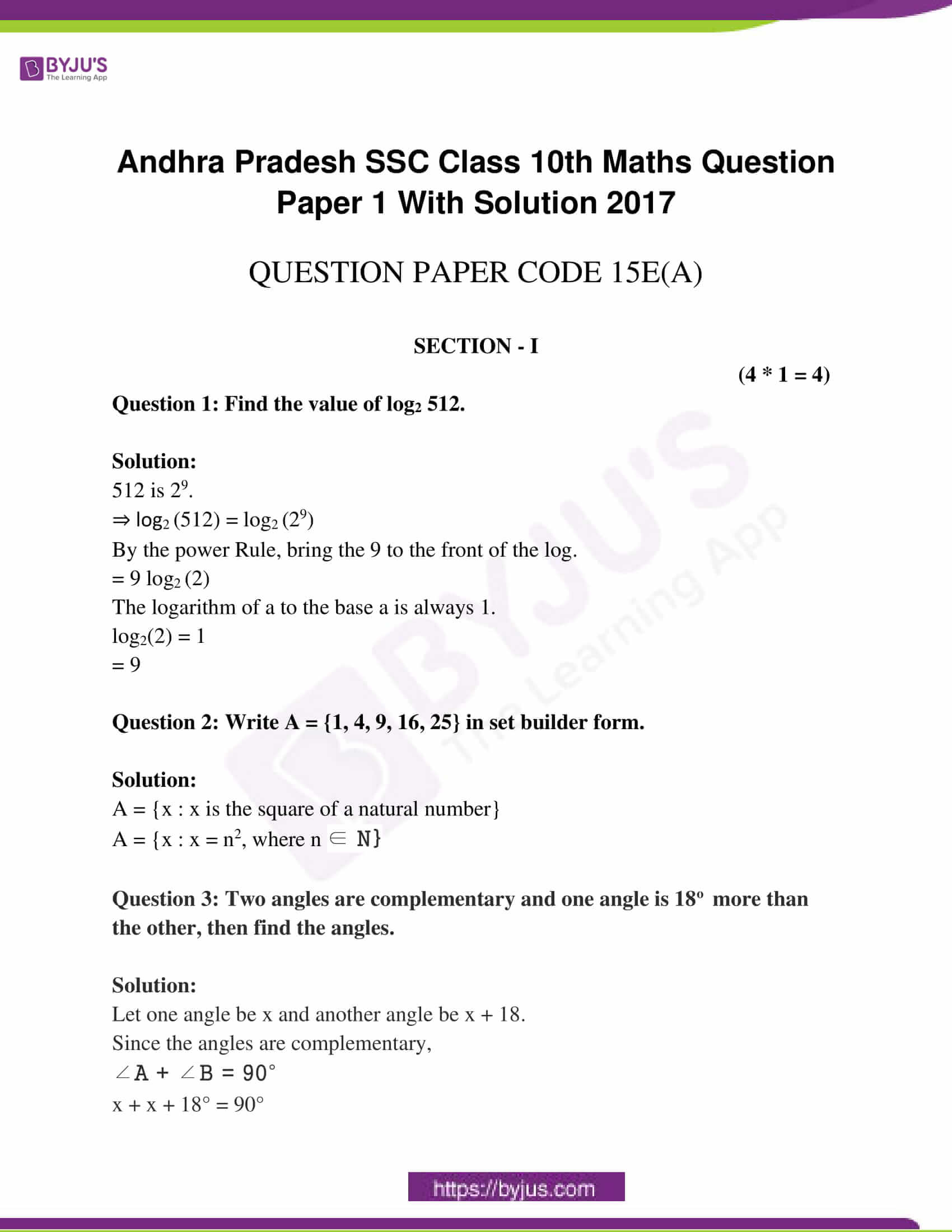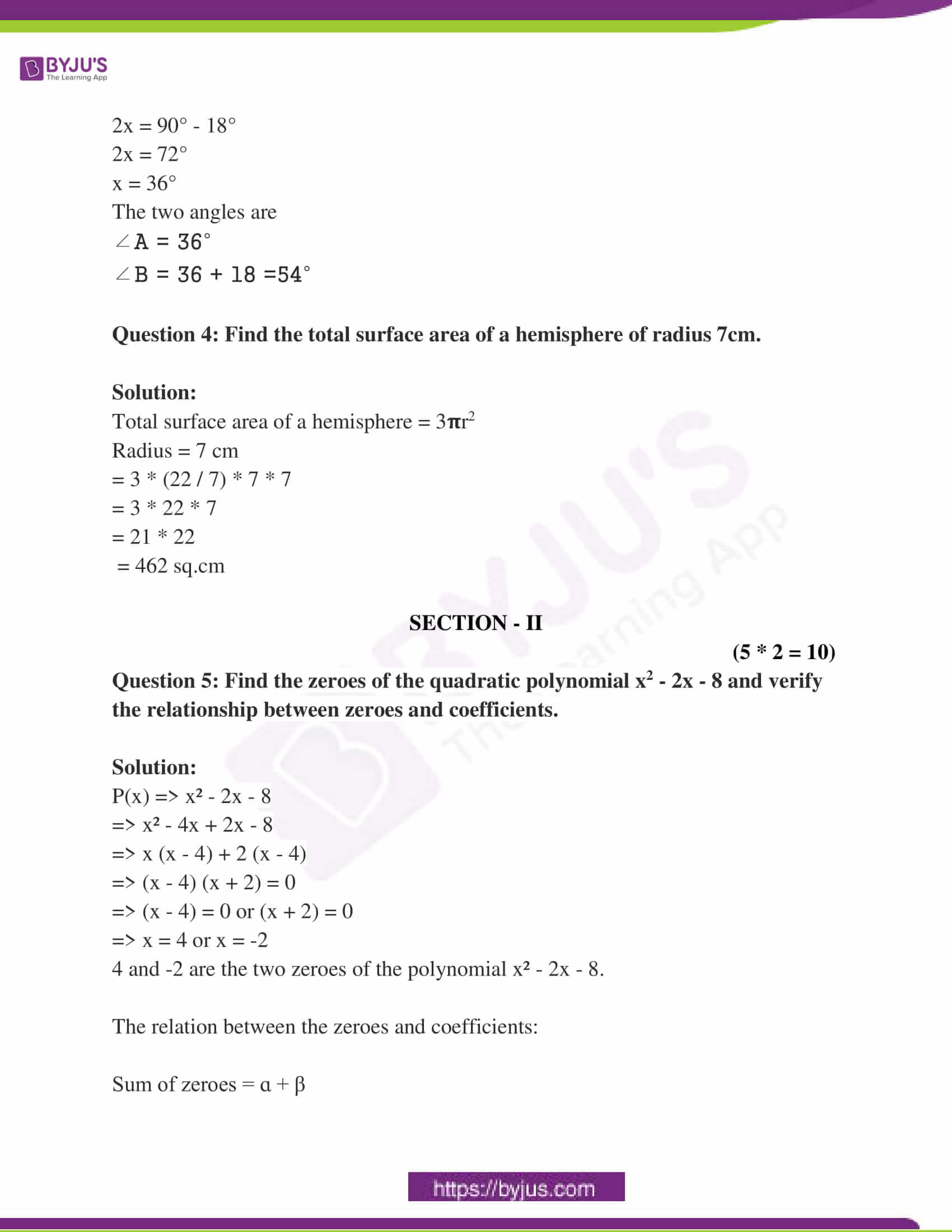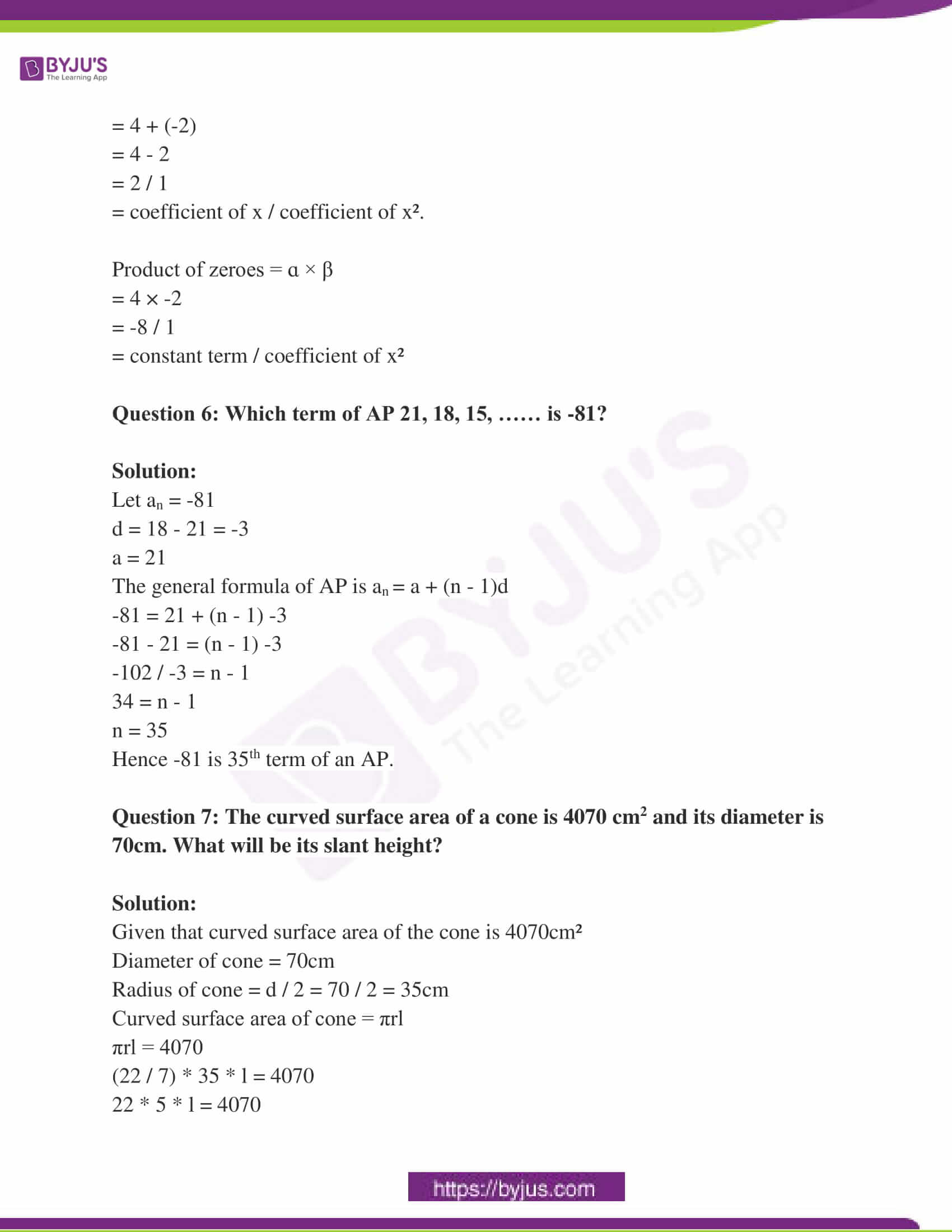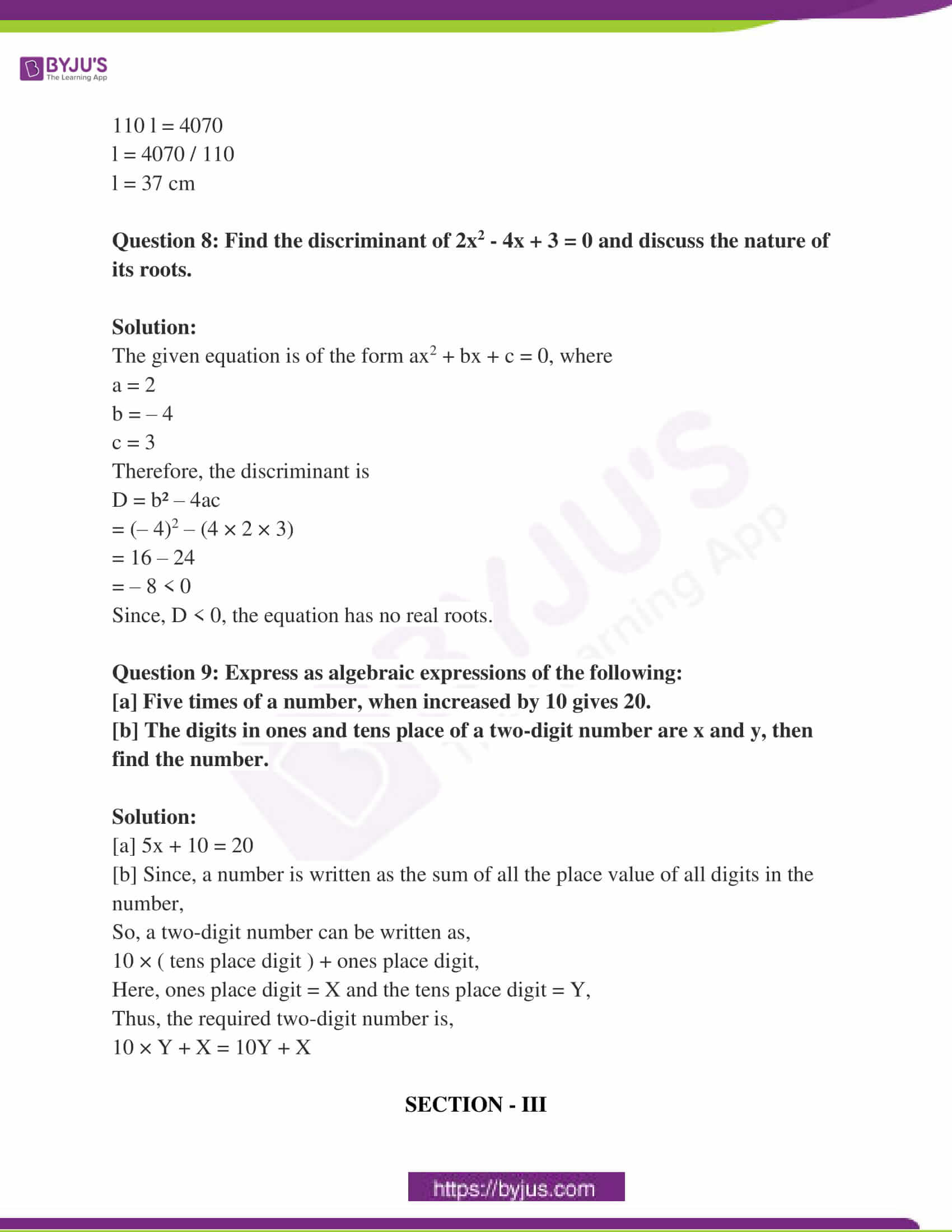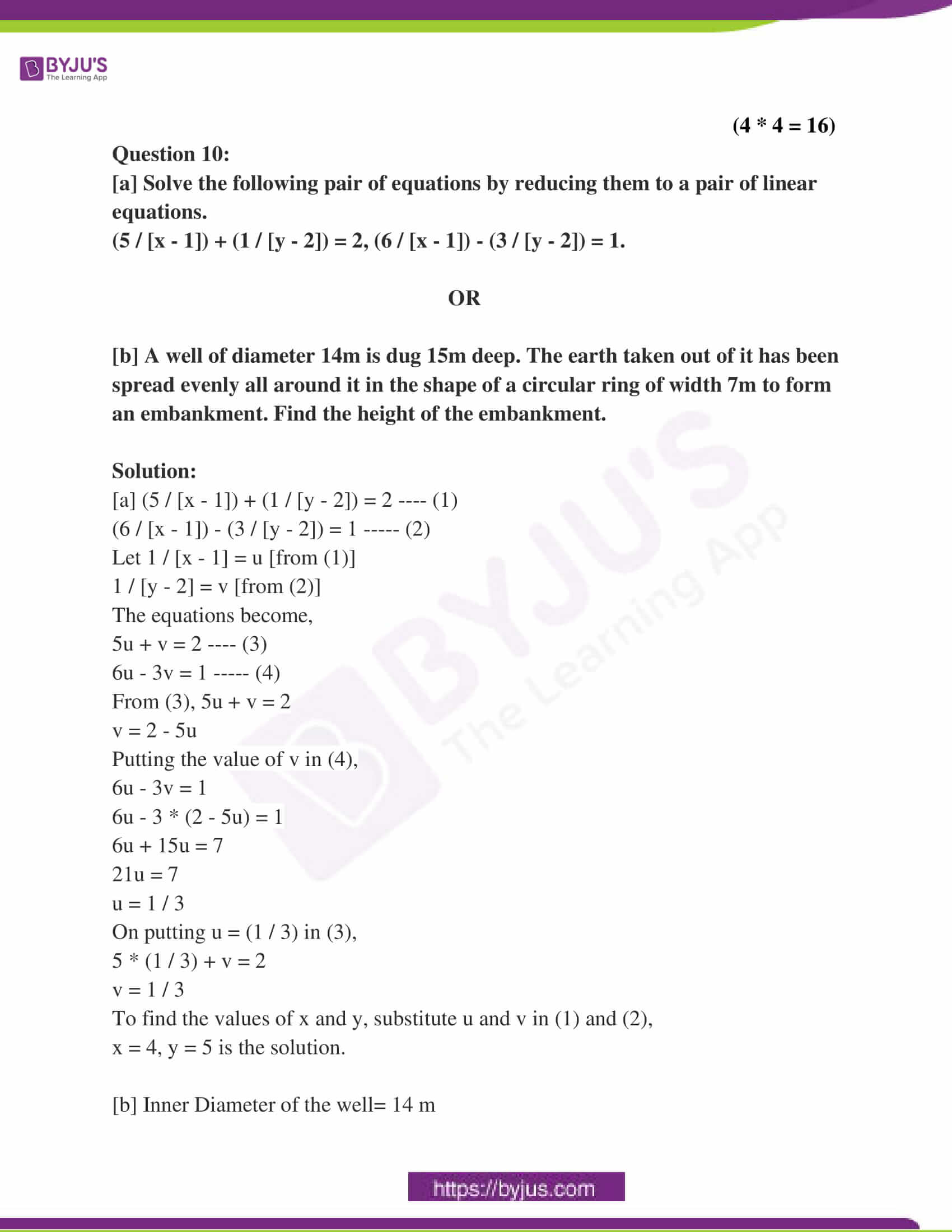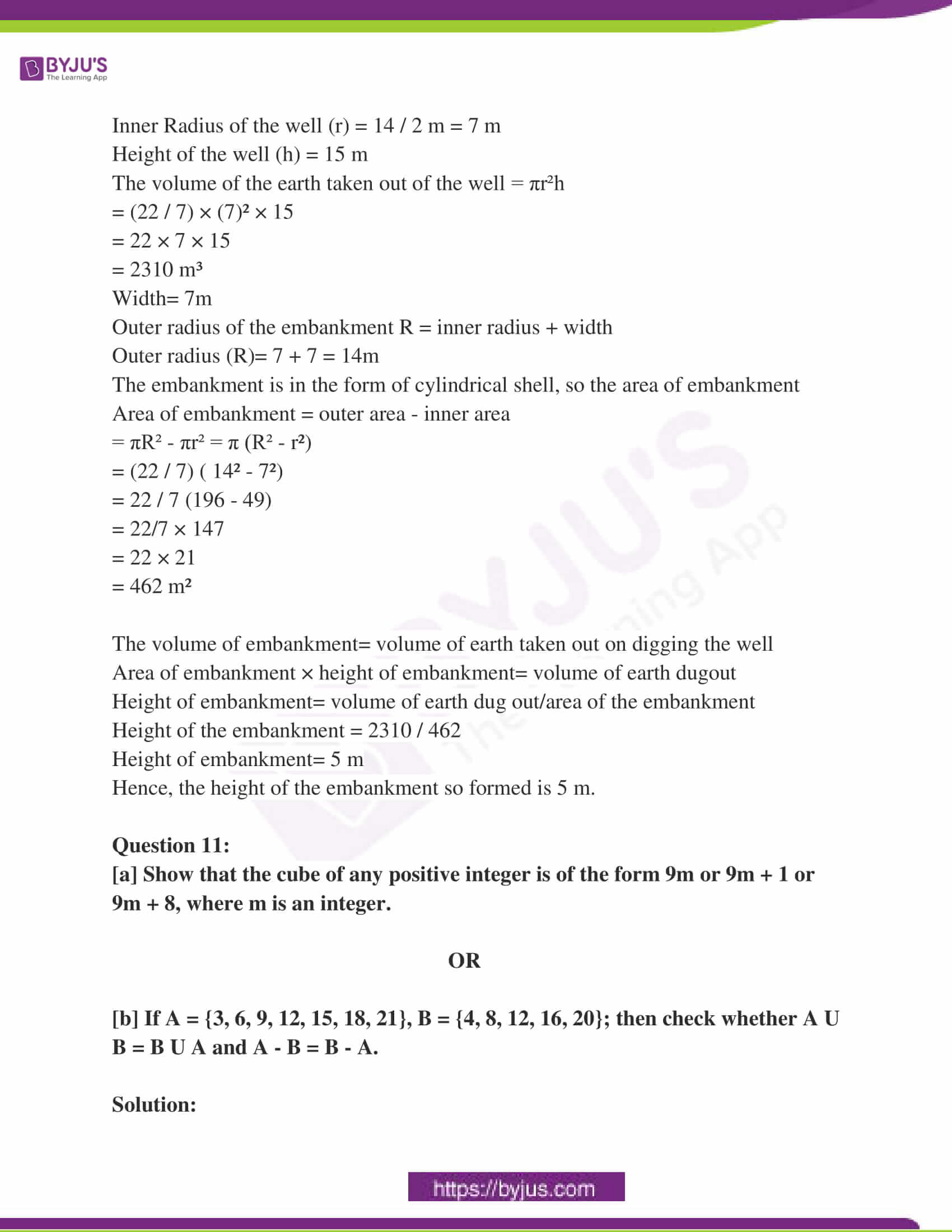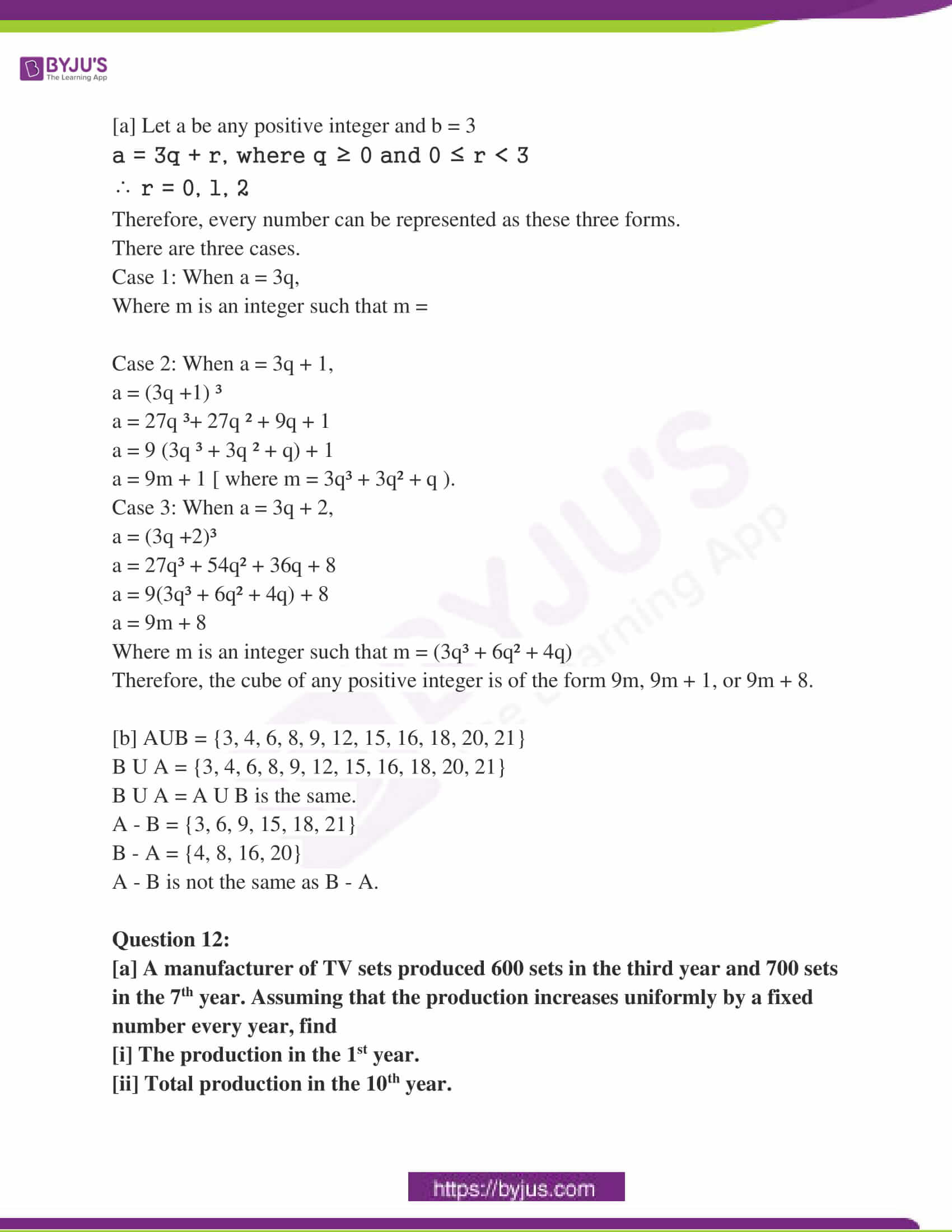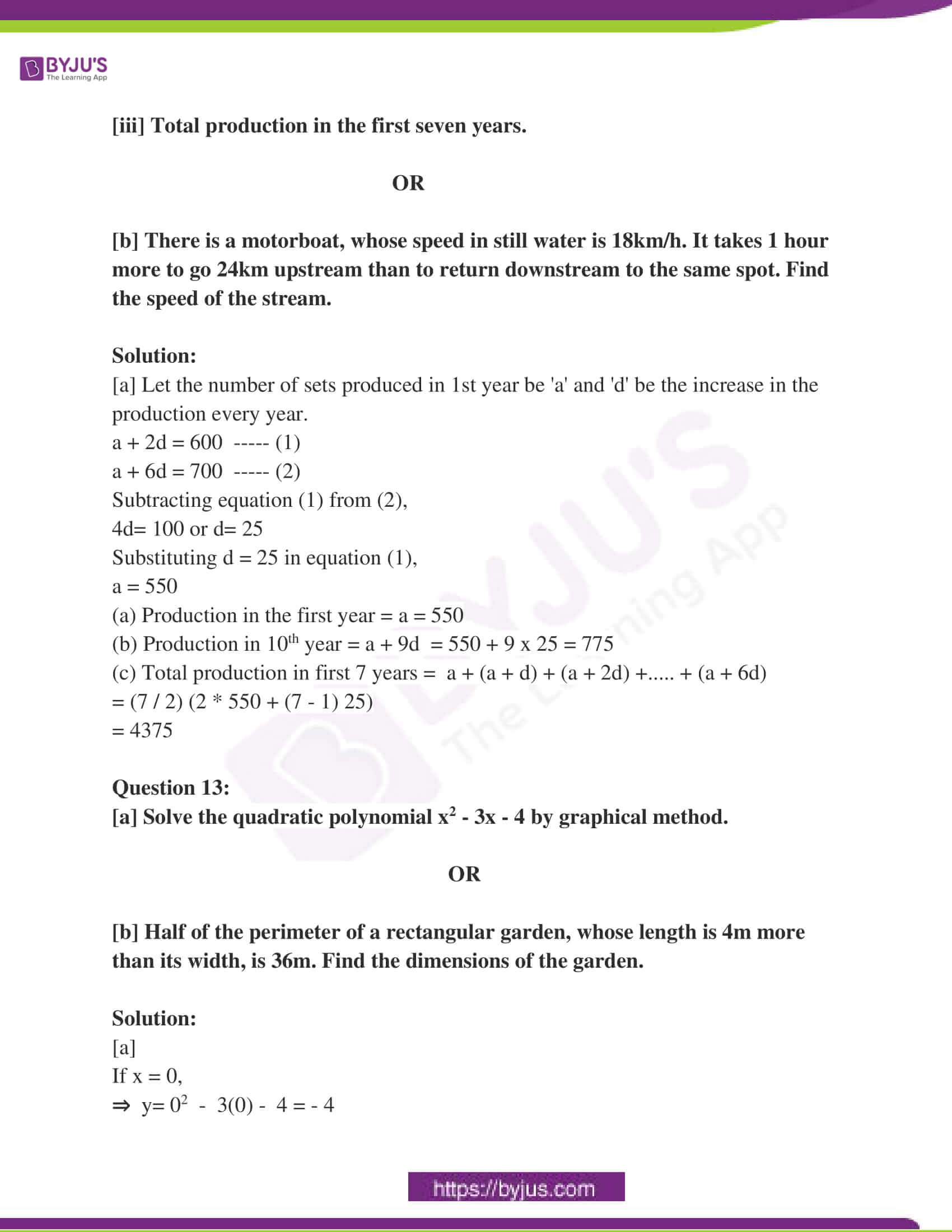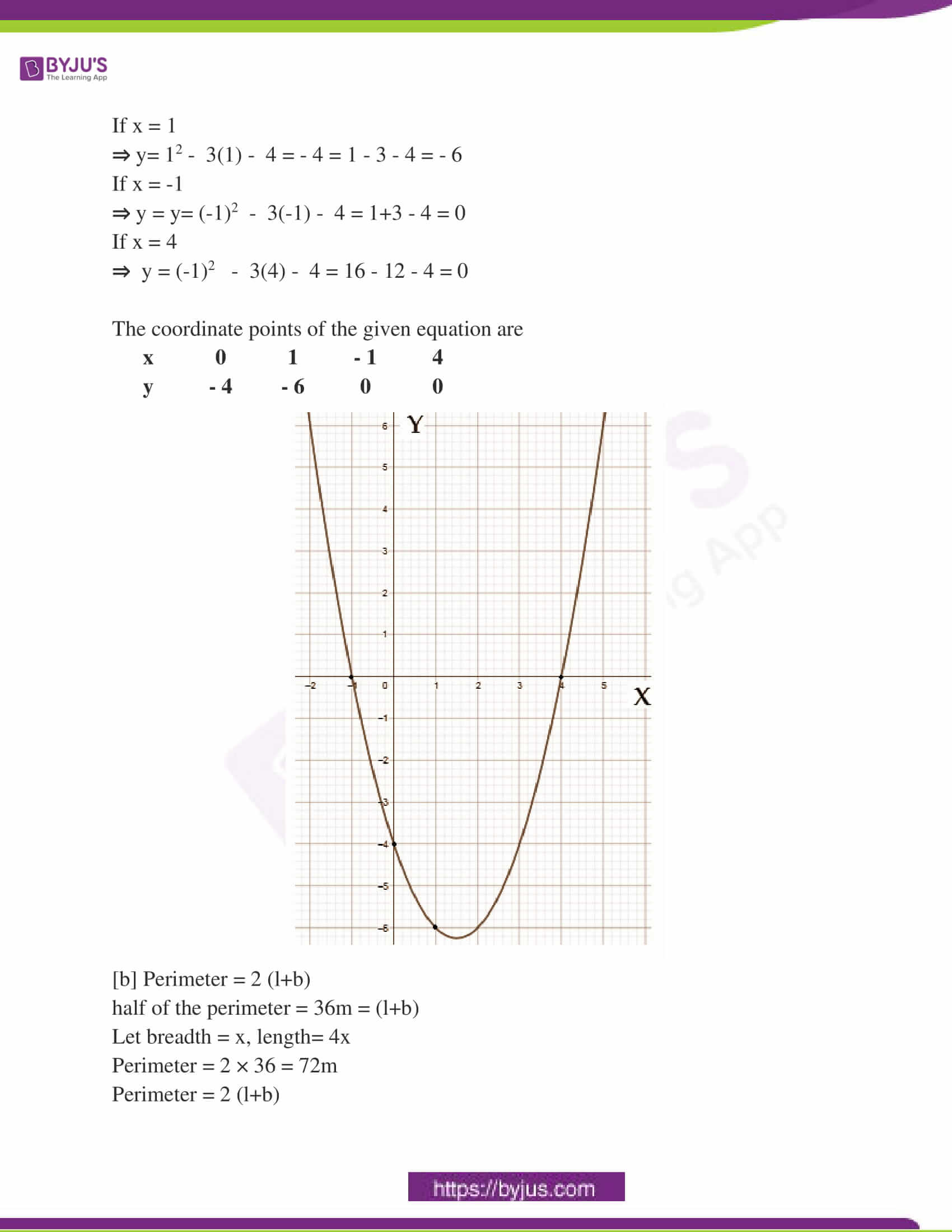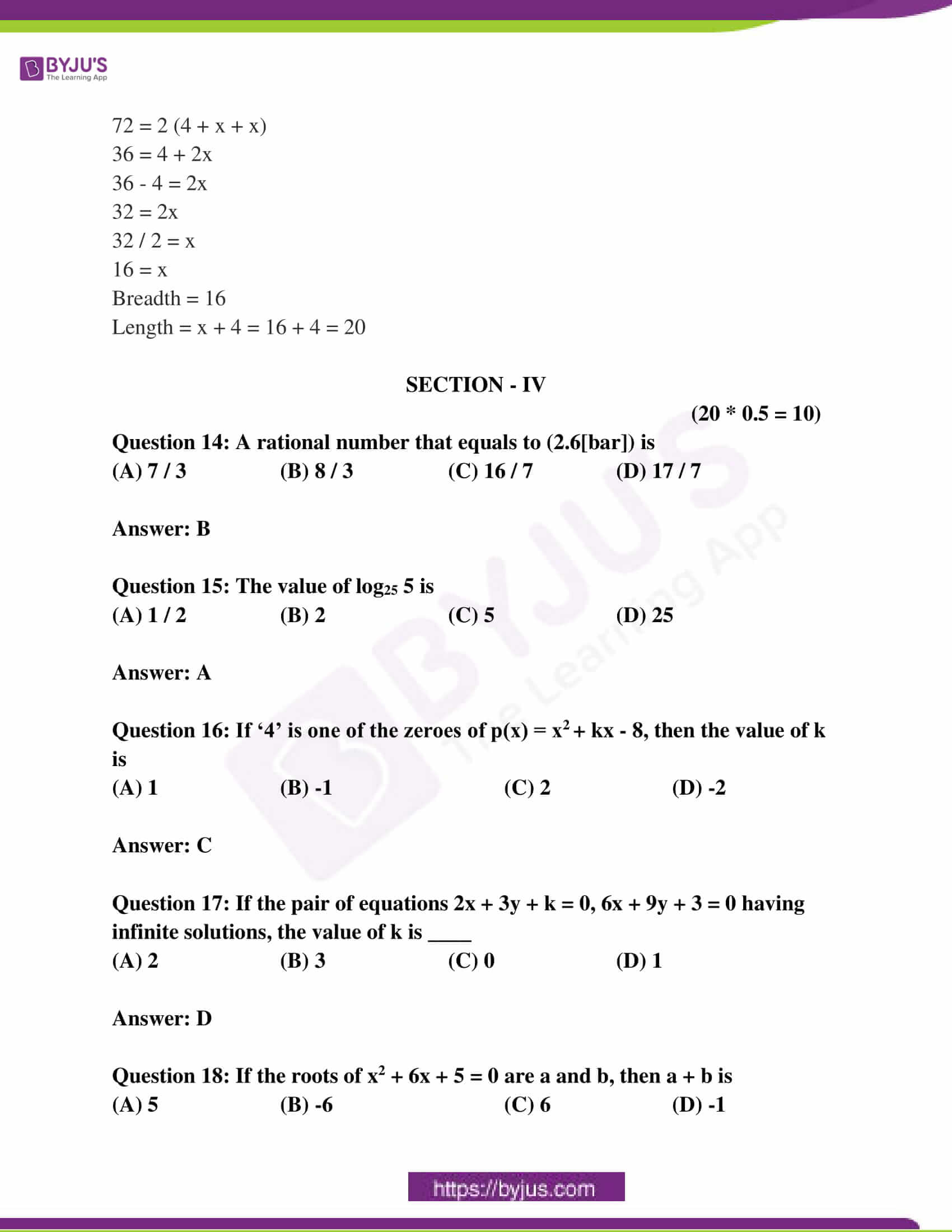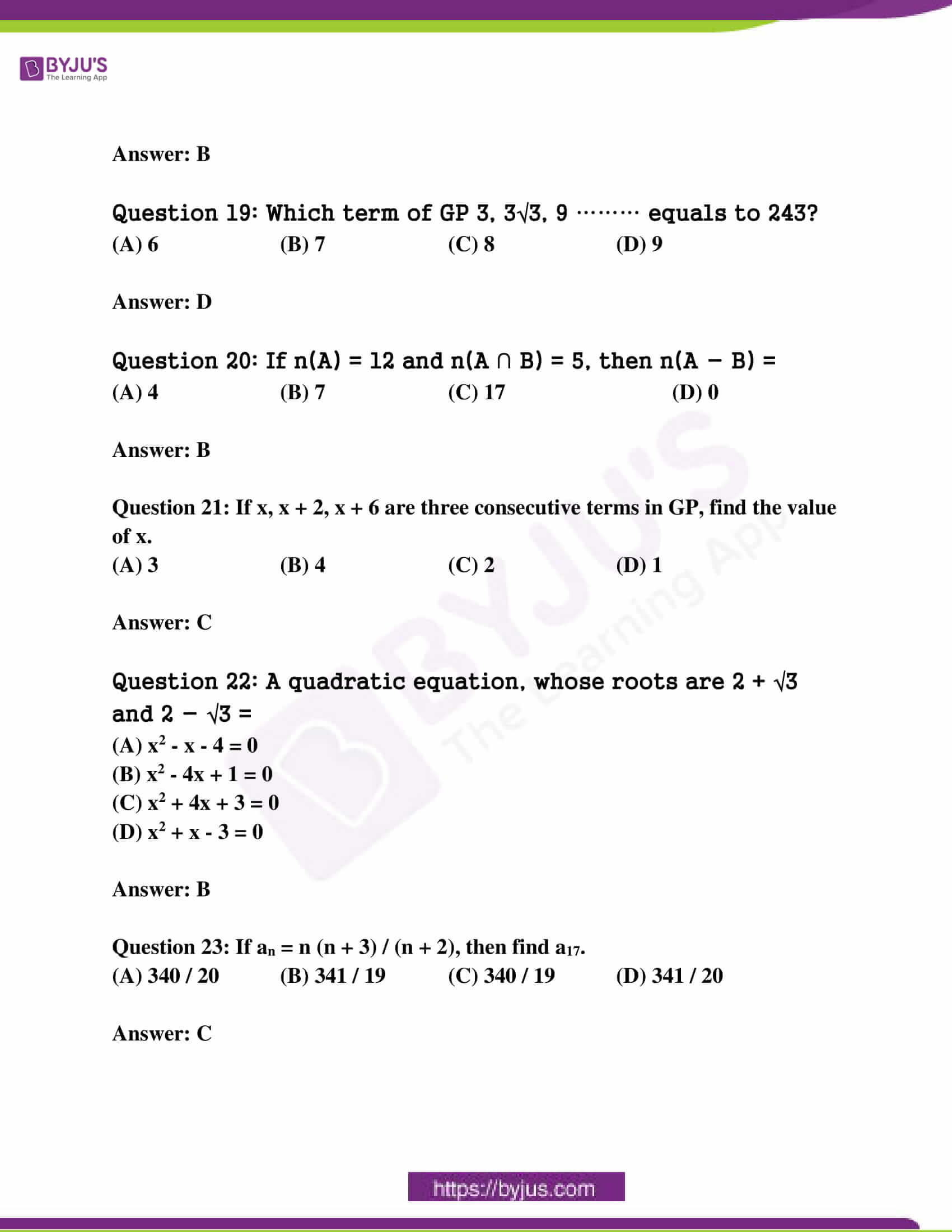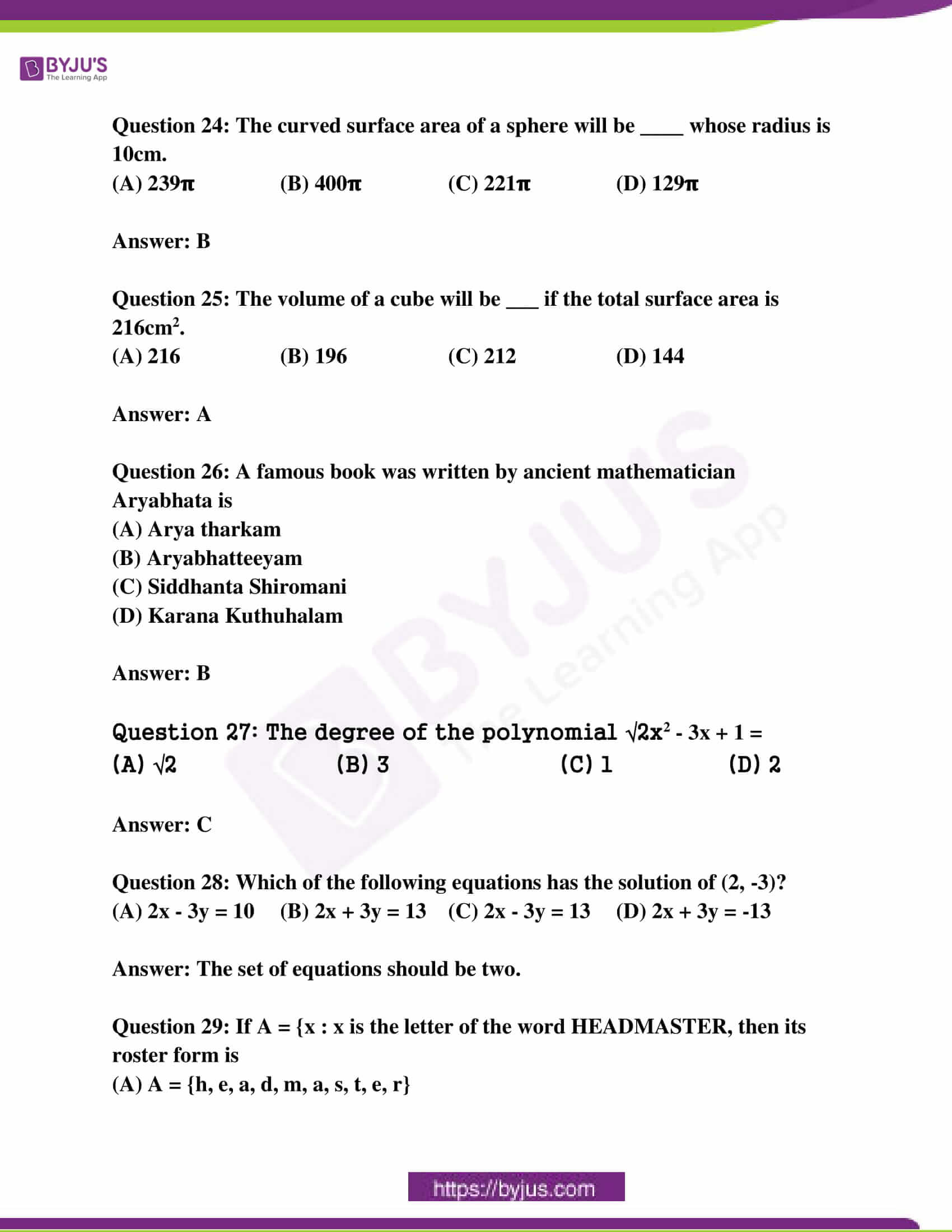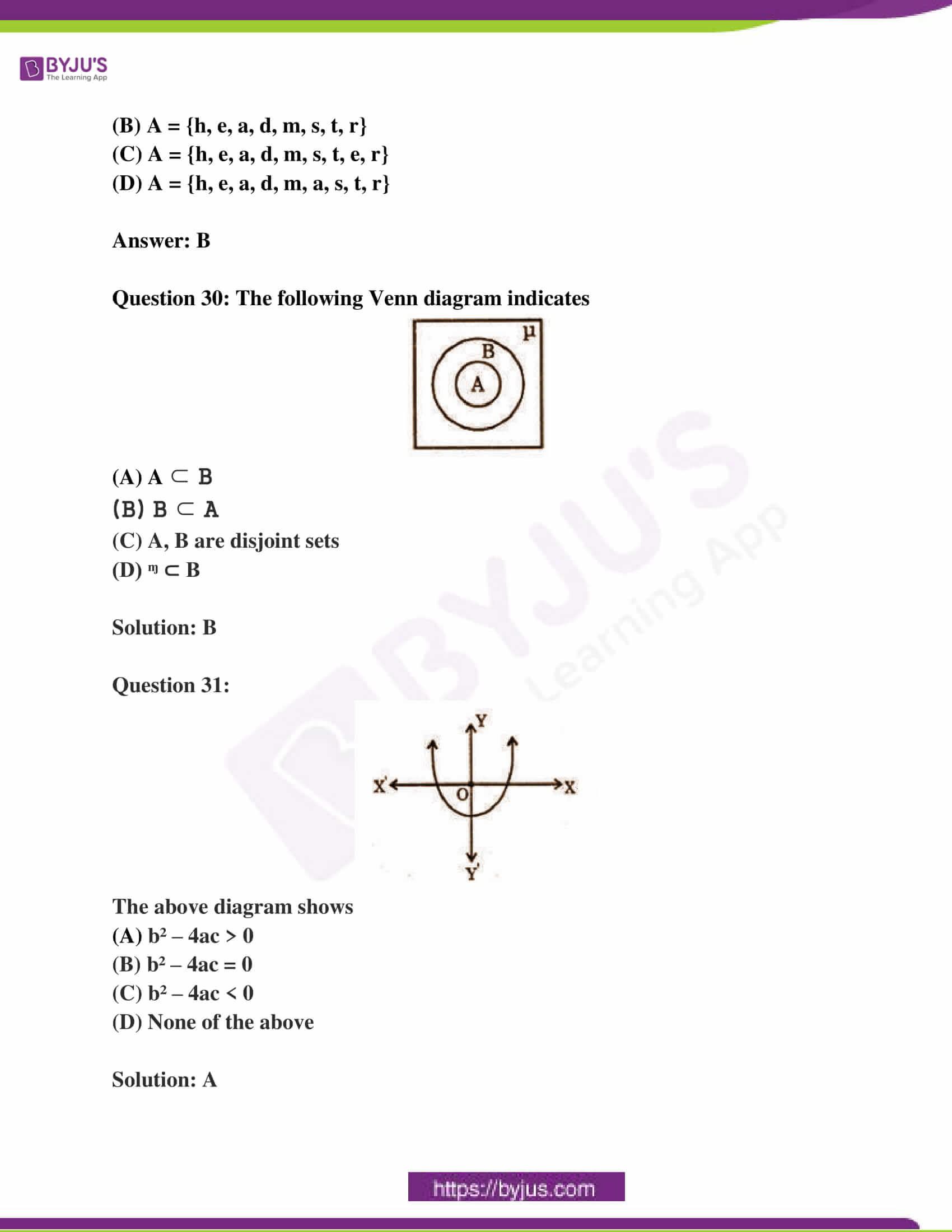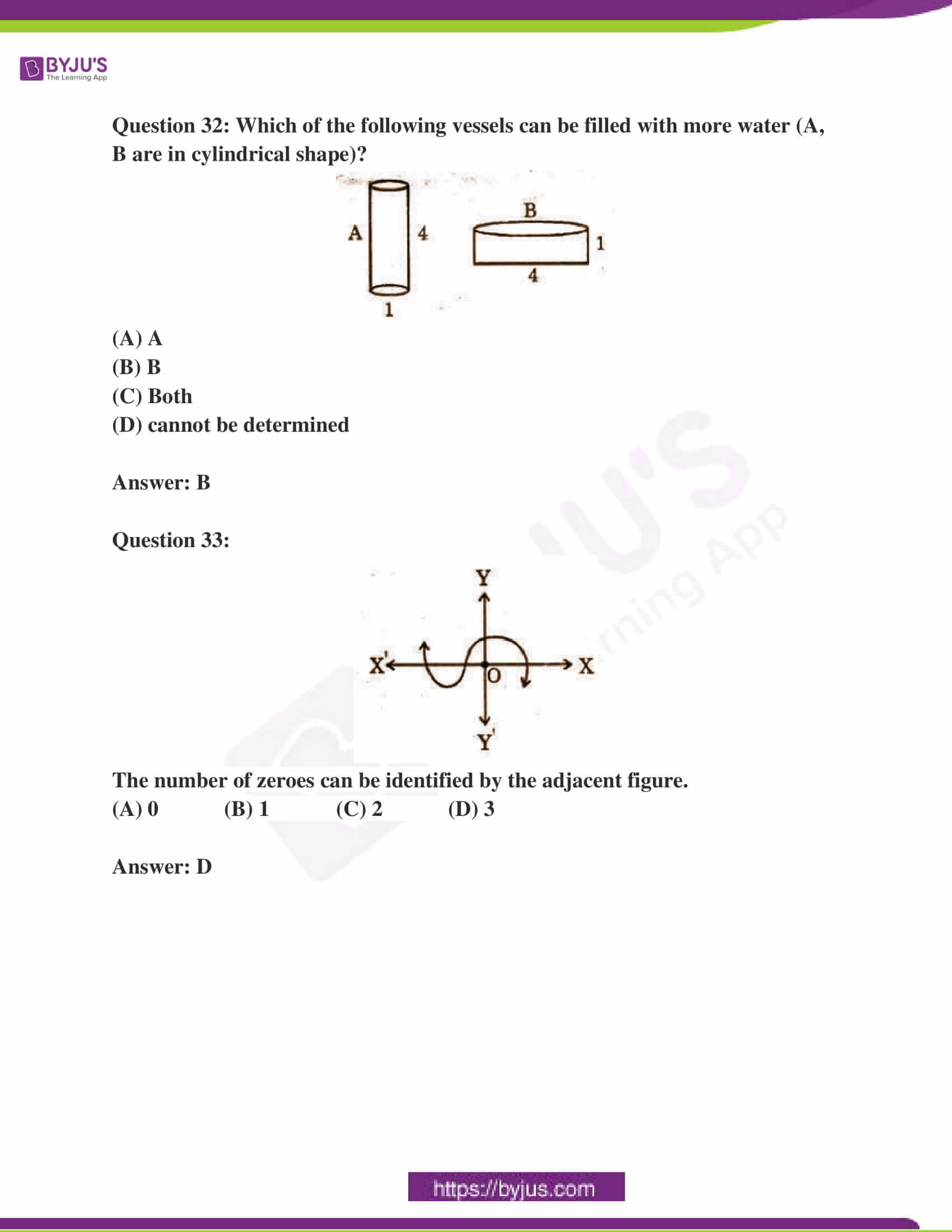SECTION – I

(4 * 1 = 4)

Question 1: Find the value of log2 512.

Solution:

512 is 29.

⇒ log2 (512) = log2 (29)

By the power Rule, bring the 9 to the front of the log.

= 9 log2 (2)

The logarithm of a to the base a is always 1.

log2(2) = 1

= 9

Question 2: Write A = {1, 4, 9, 16, 25} in set builder form.

Solution:

A = {x : x is the square of a natural number}

A = {x : x = n2, where n ∈ N}

Question 3: Two angles are complementary and one angle is 18o more than the other, then find the angles.

Solution:

Let one angle be x and another angle be x + 18.

Since the angles are complementary,

∠A + ∠B = 90°

x + x + 18° = 90°

2x = 90° – 18°

2x = 72°

x = 36°

The two angles are

∠A = 36°

∠B = 36 + 18 =54°

Question 4: Find the total surface area of a hemisphere of radius 7cm.

Solution:

Total surface area of a hemisphere = 3𝛑r2

= 3 * (22 / 7) * 7 * 7

= 3 * 22 * 7

= 21 * 22

= 462 sq.cm

SECTION – II

(5 * 2 = 10)

Question 5: Find the zeroes of the quadratic polynomial x2 – 2x – 8 and verify the relationship between zeroes and coefficients.

Solution:

P(x) => x² – 2x – 8

=> x² – 4x + 2x – 8

=> x (x – 4) + 2 (x – 4)

=> (x – 4) (x + 2) = 0

=> (x – 4) = 0 or (x + 2) = 0

=> x = 4 or x = -2

4 and -2 are the two zeroes of the polynomial x² – 2x – 8.

The relation between the zeroes and coefficients:

Sum of zeroes = ɑ + β

= 4 + (-2)

= 4 – 2

= 2 / 1

= coefficient of x / coefficient of x².

Product of zeroes = ɑ × β

= 4 × -2

= -8 / 1

= constant term / coefficient of x²

Question 6: Which term of AP 21, 18, 15, …… is -81?

Solution:

Let an = -81

d = 18 – 21 = -3

a = 21

The general formula of AP is an = a + (n – 1)d

-81 = 21 + (n – 1) -3

-81 – 21 = (n – 1) -3

-102 / -3 = n – 1

34 = n – 1

n = 35

Hence -81 is 35th term of an AP.

Question 7: The curved surface area of a cone is 4070 cm2 and its diameter is 70cm. What will be its slant height?

Solution:

Given that curved surface area of the cone is 4070cm²

Diameter of cone = 70cm

Radius of cone = d / 2 = 70 / 2 = 35cm

Curved surface area of cone = πrl

πrl = 4070

(22 / 7) * 35 * l = 4070

22 * 5 * l = 4070

110 l = 4070

l = 4070 / 110

l = 37 cm

Question 8: Find the discriminant of 2x2 – 4x + 3 = 0 and discuss the nature of its roots.

Solution:

The given equation is of the form ax2 + bx + c = 0, where

a = 2

b = – 4

c = 3

Therefore, the discriminant is

D = b² – 4ac

= (– 4)2 – (4 × 2 × 3)

= 16 – 24

= – 8 < 0

Since, D < 0, the equation has no real roots.

Question 9: Express as algebraic expressions of the following:

[a] Five times of a number, when increased by 10 gives 20.

[b] The digits in ones and tens place of a two-digit number are x and y, then find the number.

Solution:

[a] 5x + 10 = 20

[b] Since, a number is written as the sum of all the place value of all digits in the number,

So, a two-digit number can be written as,

10 × ( tens place digit ) + ones place digit,

Here, ones place digit = X and the tens place digit = Y,

Thus, the required two-digit number is,

10 × Y + X = 10Y + X

SECTION – III

(4 * 4 = 16)

Question 10:

[a] Solve the following pair of equations by reducing them to a pair of linear equations.

(5 / [x – 1]) + (1 / [y – 2]) = 2, (6 / [x – 1]) – (3 / [y – 2]) = 1.

OR

[b] A well of diameter 14m is dug 15m deep. The earth taken out of it has been spread evenly all around it in the shape of a circular ring of width 7m to form an embankment. Find the height of the embankment.

Solution:

[a] (5 / [x – 1]) + (1 / [y – 2]) = 2 —- (1)

(6 / [x – 1]) – (3 / [y – 2]) = 1 —– (2)

Let 1 / [x – 1] = u [from (1)]

1 / [y – 2] = v [from (2)]

The equations become,

5u + v = 2 —- (3)

6u – 3v = 1 —– (4)

From (3), 5u + v = 2

v = 2 – 5u

Putting the value of v in (4),

6u – 3v = 1

6u – 3 * (2 – 5u) = 1

6u + 15u = 7

21u = 7

u = 1 / 3

On putting u = (1 / 3) in (3),

5 * (1 / 3) + v = 2

v = 1 / 3

To find the values of x and y, substitute u and v in (1) and (2),

x = 4, y = 5 is the solution.

[b] Inner Diameter of the well= 14 m

Inner Radius of the well (r) = 14 / 2 m = 7 m

Height of the well (h) = 15 m

The volume of the earth taken out of the well = πr²h

= (22 / 7) × (7)² × 15

= 22 × 7 × 15

= 2310 m³

Width= 7m

Outer radius (R)= 7 + 7 = 14m

The embankment is in the form of cylindrical shell, so the area of embankment

Area of embankment = outer area – inner area

= πR² – πr² = π (R² – r²)

= (22 / 7) ( 14² – 7²)

= 22 / 7 (196 – 49)

= 22/7 × 147

= 22 × 21

= 462 m²

The volume of embankment= volume of earth taken out on digging the well

Area of embankment × height of embankment= volume of earth dugout

Height of embankment= volume of earth dug out/area of the embankment

Height of the embankment = 2310 / 462

Height of embankment= 5 m

Hence, the height of the embankment so formed is 5 m.

Question 11:

[a] Show that the cube of any positive integer is of the form 9m or 9m + 1 or 9m + 8, where m is an integer.

OR

[b] If A = {3, 6, 9, 12, 15, 18, 21}, B = {4, 8, 12, 16, 20}; then check whether A U B = B U A and A – B = B – A.

Solution:

[a] Let a be any positive integer and b = 3

a = 3q + r, where q ≥ 0 and 0 ≤ r < 3

∴ r = 0, 1, 2

Therefore, every number can be represented as these three forms.

There are three cases.

Case 1: When a = 3q,

Where m is an integer such that m =

Case 2: When a = 3q + 1,

a = (3q +1) ³

a = 27q ³+ 27q ² + 9q + 1

a = 9 (3q ³ + 3q ² + q) + 1

a = 9m + 1 [ where m = 3q³ + 3q² + q ).

Case 3: When a = 3q + 2,

a = (3q +2)³

a = 27q³ + 54q² + 36q + 8

a = 9(3q³ + 6q² + 4q) + 8

a = 9m + 8

Where m is an integer such that m = (3q³ + 6q² + 4q)

Therefore, the cube of any positive integer is of the form 9m, 9m + 1, or 9m + 8.

[b] AUB = {3, 4, 6, 8, 9, 12, 15, 16, 18, 20, 21}

B U A = {3, 4, 6, 8, 9, 12, 15, 16, 18, 20, 21}

B U A = A U B is the same.

A – B = {3, 6, 9, 15, 18, 21}

B – A = {4, 8, 16, 20}

A – B is not the same as B – A.

Question 12:

[a] A manufacturer of TV sets produced 600 sets in the third year and 700 sets in the 7th year. Assuming that the production increases uniformly by a fixed number every year, find

[i] The production in the 1st year.

[ii] Total production in the 10th year.

[iii] Total production in the first seven years.

OR

[b] There is a motorboat, whose speed in still water is 18km/h. It takes 1 hour more to go 24km upstream than to return downstream to the same spot. Find the speed of the stream.

Solution:

[a] Let the number of sets produced in 1st year be ‘a’ and ‘d’ be the increase in the production every year.

a + 2d = 600 —– (1)

a + 6d = 700 —– (2)

Subtracting equation (1) from (2),

4d= 100 or d= 25

Substituting d = 25 in equation (1),

a = 550

(a) Production in the first year = a = 550

(b) Production in 10th year = a + 9d = 550 + 9 x 25 = 775

(c) Total production in first 7 years = a + (a + d) + (a + 2d) +….. + (a + 6d)

= (7 / 2) (2 * 550 + (7 – 1) 25)

= 4375

Question 13:

[a] Solve the quadratic polynomial x2 – 3x – 4 by graphical method.

OR

[b] Half of the perimeter of a rectangular garden, whose length is 4m more than its width, is 36m. Find the dimensions of the garden.

Solution:

[a]

If x = 0,

y= 02 – 3(0) – 4 = – 4

If x = 1

y= 12 – 3(1) – 4 = – 4 = 1 – 3 – 4 = – 6

If x = -1

y = y= (-1)2 – 3(-1) – 4 = 1+3 – 4 = 0

If x = 4

y = ​​​​​​​(-1)2 – 3(4) – 4 = 16 – 12 – 4 = 0

The coordinate points of the given equation are

 x 0 1 – 1 4 y – 4 – 6 0 0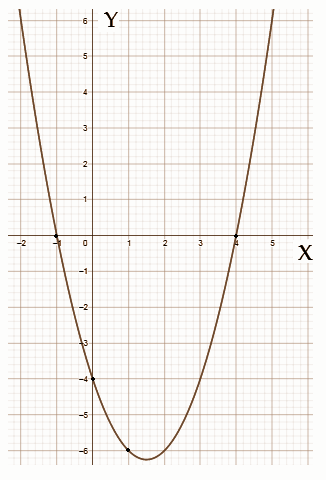[b] Perimeter = 2 (l+b)

half of the perimeter = 36m = (l+b)

Let breadth = x, length= 4x

Perimeter = 2 × 36 = 72m

Perimeter = 2 (l+b)

72 = 2 (4 + x + x)

36 = 4 + 2x

36 – 4 = 2x

32 = 2x

32 / 2 = x

16 = x

Length = x + 4 = 16 + 4 = 20

SECTION – IV

(20 * 0.5 = 10)

Question 14: A rational number that equals to (2.6[bar]) is

(A) 7 / 3 (B) 8 / 3 (C) 16 / 7 (D) 17 / 7

Question 15: The value of log25 5 is

(A) 1 / 2 (B) 2 (C) 5 (D) 25

Question 16: If ‘4’ is one of the zeroes of p(x) = x2 + kx – 8, then the value of k is

(A) 1 (B) -1 (C) 2 (D) -2

Question 17: If the pair of equations 2x + 3y + k = 0, 6x + 9y + 3 = 0 having infinite solutions, the value of k is ____

(A) 2 (B) 3 (C) 0 (D) 1

Question 18: If the roots of x2 + 6x + 5 = 0 are a and b, then a + b is

(A) 5 (B) -6 (C) 6 (D) -1

Question 19: Which term of GP 3, 3√3, 9 ……… equals to 243?

(A) 6 (B) 7 (C) 8 (D) 9

Question 20: If n(A) = 12 and n(A ∩ B) = 5, then n(A – B) =

(A) 4 (B) 7 (C) 17 (D) 0

Question 21: If x, x + 2, x + 6 are three consecutive terms in GP, find the value of x.

(A) 3 (B) 4 (C) 2 (D) 1

Question 22: A quadratic equation, whose roots are 2 + √3 and 2 – √3 =

(A) x2 – x – 4 = 0

(B) x2 – 4x + 1 = 0

(C) x2 + 4x + 3 = 0

(D) x2 + x – 3 = 0

Question 23: If an = n (n + 3) / (n + 2), then find a17.

(A) 340 / 20 (B) 341 / 19 (C) 340 / 19 (D) 341 / 20

Question 24: The curved surface area of a sphere will be ____ whose radius is 10cm.

(A) 239𝛑 (B) 400𝛑 (C) 221𝛑 (D) 129𝛑

Question 25: The volume of a cube will be ___ if the total surface area is 216cm2.

(A) 216 (B) 196 (C) 212 (D) 144

Question 26: A famous book written by ancient mathematician Aryabhata is

(A) Arya tharkam

(B) Aryabhatteeyam

(C) Siddhanta Shiromani

(D) Karana Kuthuhalam

Question 27: The degree of the polynomial √2x2 – 3x + 1 =

(A) √2 (B) 3 (C) 1 (D) 2

Question 28: Which of the following equations has the solution of (2, -3)?

(A) 2x – 3y = 10 (B) 2x + 3y = 13 (C) 2x – 3y = 13 (D) 2x + 3y = -13

Answer: The set of equations should be two.

Question 29: If A = {x : x is the letter of the word HEADMASTER, then its roster form is

(A) A = {h, e, a, d, m, a, s, t, e, r}

(B) A = {h, e, a, d, m, s, t, r}

(C) A = {h, e, a, d, m, s, t, e, r}

(D) A = {h, e, a, d, m, a, s, t, r}

Question 30: The following Venn diagram indicates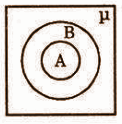(A) A ⊂ B

(B) B ⊂ A

(C) A, B are disjoint sets

(D) ᶬ ⊂ B

Solution: B

Question 31: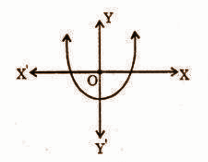The above diagram shows

(A) b² – 4ac > 0

(B) b² – 4ac = 0

(C) b² – 4ac < 0

(D) None of the above

Solution: A

Question 32: Which of the following vessels can be filled with more water (A, B are in cylindrical shape)?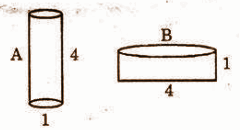(A) A

(B) B

(C) Both

(D) cannot be determined

Question 33: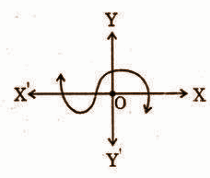The number of zeroes can be identified by the adjacent figure.

(A) 0 (B) 1 (C) 2 (D) 3